## Using Two Samples

To compare two means or two proportions, one works with two groups.

### Learning Objectives

Distinguish between independent and matched pairs in terms of hypothesis tests comparing two groups.

### Key Takeaways

#### Key Points

• The groups are classified either as independent or matched pairs.
• Independent groups mean that the two samples taken are independent, that is, sample values selected from one population are not related in any way to sample values selected from the other population.
• Matched pairs consist of two samples that are dependent.

#### Key Terms

• matched pair: A data set of two groups consisting of two samples that are dependent.
• independent group: A statistical group of random variables that has the same probability distribution as the others, and that are all mutually independent.

Studies often compare two groups. For example, researchers are interested in the effect aspirin has in preventing heart attacks. Over the last few years, newspapers and magazines have reported about various aspirin studies involving two groups. Typically, one group is given aspirin and the other group is given a placebo. Then, the heart attack rate is studied over several years.

There are other situations that deal with the comparison of two groups. For example, studies compare various diet and exercise programs. Politicians compare the proportion of individuals from different income brackets who might vote for them. Students are interested in whether SAT or GRE preparatory courses really help raise their scores.

In the previous section, we explained how to conduct hypothesis tests on single means and single proportions. We will expand upon that in this section. You will compare two means or two proportions to each other. The general procedure is still the same, just expanded.

To compare two means or two proportions, one works with two groups. The groups are classified either as independent or matched pairs. Independent groups mean that the two samples taken are independent, that is, sample values selected from one population are not related in any way to sample values selected from the other population. Matched pairs consist of two samples that are dependent. The parameter tested using matched pairs is the population mean (see ). The parameters tested using independent groups are either population means or population proportions.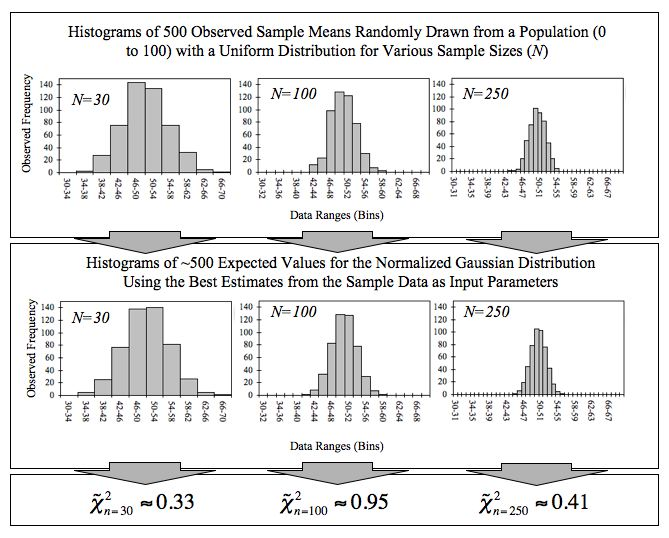The Population Mean: This image shows a series of histograms for a large number of sample means taken from a population. Recall that as more sample means are taken, the closer the mean of these means will be to the population mean. In this section, we explore hypothesis testing of two independent population means (and proportions) and also tests for paired samples of population means.

To conclude, this section deals with the following hypothesis tests:

• Tests of two independent population means
• Tests of two independent population proportions
• Tests of matched or paired samples (necessarily a test of the population mean)

## Comparing Two Independent Population Means

To compare independent samples, both populations are normally distributed with the population means and standard deviations unknown.

### Learning Objectives

Outline the mechanics of a hypothesis test comparing two independent population means.

### Key Takeaways

#### Key Points

• Very different means can occur by chance if there is great variation among the individual samples.
• In order to account for the variation, we take the difference of the sample means and divide by the standard error in order to standardize the difference.
• Because we do not know the population standard deviations, we estimate them using the two sample standard deviations from our independent samples.

#### Key Terms

• degrees of freedom (df): The number of objects in a sample that are free to vary.
• t-score: A score utilized in setting up norms for standardized tests; obtained by linearly transforming normalized standard scores.

Independent samples are simple random samples from two distinct populations. To compare these random samples, both populations are normally distributed with the population means and standard deviations unknown unless the sample sizes are greater than 30. In that case, the populations need not be normally distributed.

The comparison of two population means is very common. The difference between the two samples depends on both the means and the standard deviations. Very different means can occur by chance if there is great variation among the individual samples. In order to account for the variation, we take the difference of the sample means,

$\bar { { \text{X} }_{ 1 } } -\bar { { \text{X} }_{ 2 } }$

and divide by the standard error (shown below) in order to standardize the difference. The result is a $\text{t}$score test statistic (also shown below).

Because we do not know the population standard deviations, we estimate them using the two sample standard deviations from our independent samples. For the hypothesis test, we calculate the estimated standard deviation, or standard error, of the difference in sample means,

$\bar { { \text{X} }_{ 1 } } -\bar { { \text{X} }_{ 2 } }$.

The standard error is:

$\displaystyle \sqrt { \frac { { \text{S} }_{ 1 }^{ 2 } }{ { \text{n} }_{ 1 } } +\frac { { \text{S} }_{ 2 }^{ 2 } }{ { \text{n} }_{ 2 } } }$.

The test statistic ($\text{t}$-score) is calculated as follows:

$\dfrac { (\bar { { \text{X} }_{ 1 } } -\bar { { \text{X} }_{ 2 } } )-({ \mu }_{ 1 }-{ \mu }_{ 2 }) }{ \sqrt { \dfrac { { \text{S} }_{ 1 }^{ 2 } }{ { \text{n} }_{ 1 } } +\dfrac { { \text{S} }_{ 2 }^{ 2 } }{ { \text{n} }_{ 2 } } } }$.

The degrees of freedom ($\text{df}$) is a somewhat complicated calculation. The $\text{df}$s are not always a whole number. The test statistic calculated above is approximated by the student’s-$\text{t}$ distribution with $\text{df}$s as follows:

$\displaystyle df=\frac { { \left( \frac { { \text{S} }_{ 1 }^{ 2 } }{ { \text{n} }_{ 1 } } +\frac { { \text{S} }_{ 2 }^{ 2 } }{ { \text{n} }_{ 2 } } \right) }^{ 2 } }{ \left[ \left( \frac { 1 }{ { \text{n} }_{ 1 } } -1 \right) \cdot { \left( \frac { { \text{S} }_{ 1 }^{ 2 } }{ { \text{n} }_{ 1 } } \right) }^{ 2 }+\left( \frac { 1 }{ { \text{n} }_{ 2 } } -1 \right) \cdot { \left( \frac { { \text{S} }_{ 2 }^{ 2 } }{ { \text{n} }_{ 2 } } \right) }^{ 2 } \right] }$

Note that it is not necessary to compute this by hand. A calculator or computer easily computes it.

### Example

The average amount of time boys and girls ages 7 through 11 spend playing sports each day is believed to be the same. An experiment is done, data is collected, resulting in the table below. Both populations have a normal distribution.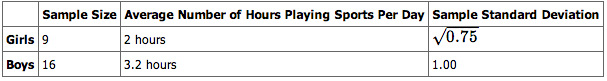Independent Sample Table 1: This table lays out the parameters for our example.

Is there a difference in the mean amount of time boys and girls ages 7 through 11 play sports each day? Test at the 5% level of significance.

### Solution

The population standard deviations are not known. Let $\text{g}$ be the subscript for girls and $\text{b}$ be the subscript for boys. Then, $\mu_\text{g}$ is the population mean for girls and $\mu_\text{b}$ is the population mean for boys. This is a test of two independent groups, two population means.

The random variable: $\bar { { \text{X} }_{ \text{g} } } -\bar { { \text{X} }_{ \text{b} } }$ is the difference in the sample mean amount of time girls and boys play sports each day.

$\text{H}_0: \mu_\text{g} = \mu_{\text{bg}-\text{b}} = 0$

$\text{H}_\text{a}: \mu_\text{g} \neq \mu_{\text{bg}-\text{b}} \neq 0$

The words “the same” tell you $\text{H}_0$ has an “=”. Since there are no other words to indicate $\text{H}_\text{a}$, then assume “is different.” This is a two-tailed test.

Distribution for the test: Use $\text{t}_{\text{df}}$ where $\text{df}$ is calculated using the $\text{df}$ formula for independent groups, two population means. Using a calculator, $\text{df}$ is approximately 18.8462.

Calculate the $\text{p}$-value using a student’s-$\text{t}$ distribution: $\text{p}\text{-value} = 0.0054$

Graph: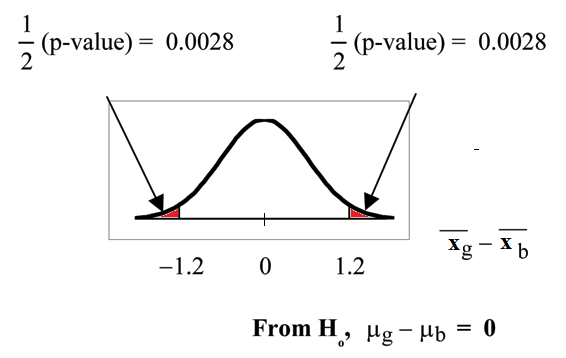Graph for Example: This image shows the graph for the $\text{p}$-values in our example.

${ \text{s} }_{ \text{g} }=\sqrt { 0.75 }$

${ \text{s} }_{ \text{b} }=1$

so, $\bar { { \text{X} }_{ \text{g} } } -\bar { { \text{X} }_{ \text{b} } }=2-3.1=-1.2$

Half the $\text{p}$-value is below $-1.2$ and half is above 1.2.

Make a decision: Since $\alpha > \text{p}\text{-value}$, reject $\text{H}_0$. This means you reject $\mu_\text{g} = \mu_\text{b}$. The means are different.

Conclusion: At the 5% level of significance, the sample data show there is sufficient evidence to conclude that the mean number of hours that girls and boys aged 7 through 11 play sports per day is different (the mean number of hours boys aged 7 through 11 play sports per day is greater than the mean number of hours played by girls OR the mean number of hours girls aged 7 through 11 play sports per day is greater than the mean number of hours played by boys).

## Comparing Two Independent Population Proportions

If two estimated proportions are different, it may be due to a difference in the populations or it may be due to chance.

### Learning Objectives

Demonstrate how a hypothesis test can help determine if a difference in estimated proportions reflects a difference in population proportions.

### Key Takeaways

#### Key Points

• Comparing two proportions (e.g., comparing two means) is common.
• A hypothesis test can help determine if a difference in the estimated proportions reflects a difference in the population proportions.
• The difference of two proportions follows an approximate normal distribution.
• Generally, the null hypothesis states that the two proportions are the same.

#### Key Terms

• random sample: a sample randomly taken from an investigated population
• independent sample: Two samples are independent as they are drawn from two different populations, and the samples have no effect on each other.

1. The two independent samples are simple random samples that are independent.
2. The number of successes is at least five and the number of failures is at least five for each of the samples.

Comparing two proportions (e.g., comparing two means) is common. If two estimated proportions are different, it may be due to a difference in the populations or it may be due to chance. A hypothesis test can help determine if a difference in the estimated proportions:

${ \text{P} }_{ \text{A} }^{ ‘ }-{ \text{P} }_{ \text{B} }^{ ‘ }$

reflects a difference in the population proportions.

The difference of two proportions follows an approximate normal distribution. Generally, the null hypothesis states that the two proportions are the same. That is, $\text{H}_0: \text{p}_\text{A} = \text{p}_\text{B}$. To conduct the test, we use a pooled proportion, $\text{p}_\text{c}$.

The pooled proportion is calculated as follows:

${ \text{p} }_{ \text{c} }=\dfrac { { \text{x} }_{ \text{A} }+{ \text{x} }_{ \text{B} } }{ { \text{n} }_{ \text{A} }+{ \text{n} }_{ \text{B} } }$

The distribution for the differences is:

$\displaystyle { \text{P} }_{ \text{A} }^{ ‘ }-{ \text{P} }_{ \text{B} }^{ ‘ }\sim \text{N}\left[ 0,\sqrt { { \text{p} }_{ \text{c} }\cdot (1-{ \text{p} }_{ \text{c} })\cdot \left( \frac { 1 }{ { \text{n} }_{ \text{A} } } +\frac { 1 }{ { \text{n} }_{ \text{B} } } \right) } \right]$.

The test statistic ($\text{z}$-score) is:

$\displaystyle \text{z}=\frac { { (\text{p} }_{ \text{A} }^{ ‘ }-{ \text{p} }_{ \text{B} }^{ ‘ })-({ \text{p} }_{ \text{A} }-{ \text{p} }_{ \text{B} }) }{ \sqrt { { \text{p} }_{ \text{c} }\cdot (1-{ \text{p} }_{ \text{c} })\cdot \left( \frac { 1 }{ { \text{n} }_{ \text{A} } } +\frac { 1 }{ { \text{n} }_{ \text{B} } } \right) } }$.

### Example

Two types of medication for hives are being tested to determine if there is a difference in the proportions of adult patient reactions. 20 out of a random sample of 200 adults given medication $\text{A}$ still had hives 30 minutes after taking the medication. 12 out of another random sample of 200 adults given medication $\text{B}$ still had hives 30 minutes after taking the medication. Test at a 1% level of significance.

Let $\text{A}$ and $\text{B}$ be the subscripts for medication $\text{A}$ and medication $\text{B}$. Then $\text{p}_\text{A}$ and $\text{p}_\text{B}$ are the desired population proportions.

Random Variable:

${ \text{P} }_{ \text{A} }^{ ‘ }-{ \text{P} }_{ \text{B} }^{ ‘ }$

is the difference in the proportions of adult patients who did not react after 30 minutes to medication $\text{A}$ and medication $\text{B}$.

$\text{H}_0: \text{p}_\text{A} = \text{p}_\text{B}\text{p}_\text{A} - \text{p}_\text{B} = 0$

$\text{H}_\text{a}: \text{p}_\text{A} \neq \text{p}_\text{B}\text{p}_\text{A} - \text{p}_\text{B} \neq 0$

The words “is a difference” tell you the test is two-tailed.

Distribution for the test: Since this is a test of two binomial population proportions, the distribution is normal:

$\displaystyle { \text{p} }_{ \text{c} }=\frac { { \text{x} }_{ \text{A} }+{ \text{x} }_{ \text{B} } }{ { \text{n} }_{ \text{A} }+{ \text{n} }_{ \text{B} } } =\frac { 20+12 }{ 200+200 } =0.08 \\ 1-{ \text{p} }_{ \text{c} }=0.92$.

Therefore:

$\displaystyle { \text{P} }_{ \text{A} }^{ ‘ }-{ \text{P} }_{ \text{B} }^{ ‘ }\sim \text{N}\left[ 0,\sqrt { (0.08\cdot (0.92)\cdot \left( \frac { 1 }{ 200 } +\frac { 1 }{ 200 } \right) } \right]$

${ \text{P} }_{ \text{A} }^{ ‘ }-{ \text{P} }_{ \text{B} }^{ ‘ }$ follows an approximate normal distribution.

Calculate the $\text{p}$-value using the normal distribution: $\text{p}\text{-value} = 0.1404$.

Estimated proportion for group $\text{A}$: $\displaystyle { \text{p} }_{ \text{A} }^{ ‘ }=\frac { { \text{x} }_{ \text{A} } }{ \text{n}_{ \text{A} } } =\frac { 20 }{ 200 } =0.1$

Estimated proportion for group $\text{B}$: $\displaystyle { \text{p} }_{ \text{B} }^{ ‘ }=\frac { { \text{x} }_{ \text{B} } }{ \text{n}_{ \text{B} } } =\frac { 12 }{ 200 } =0.06$

Graph: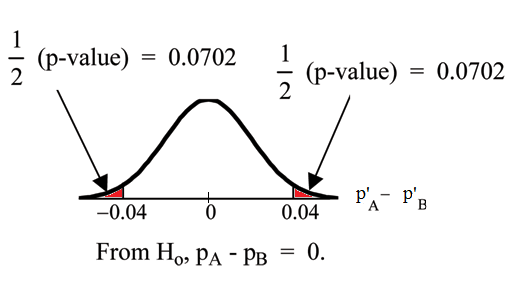$\text{p}$-Value Graph: This image shows the graph of the $\text{p}$-values in our example.

$\text{P}'_\text{A} - \text{P}'_\text{B} = 0.1 -0.06 = 0.04$.

Half the $\text{p}$-value is below $-0.04$ and half is above 0.04.

Compare $\alpha$ and the $\text{p}$-value: $\alpha = 0.01$ and the $\text{p}\text{-value}=0.1404$. $\alpha = \text{p}\text{-value}$.

Make a decision: Since $\alpha = \text{p}\text{-value}$, do not reject $\text{H}_0$.

Conclusion: At a 1% level of significance, from the sample data, there is not sufficient evidence to conclude that there is a difference in the proportions of adult patients who did not react after 30 minutes to medication $\text{A}$ and medication $\text{B}$.

## Comparing Matched or Paired Samples

In a hypothesis test for matched or paired samples, subjects are matched in pairs and differences are calculated.

### Learning Objectives

Construct a hypothesis test in which the data set is the set of differences between matched or paired samples.

### Key Takeaways

#### Key Points

• The difference between the paired samples is the target parameter.
• The population mean for the differences is tested using a Student-$\text{t}$ test for a single population mean with $\text{n}-1$ degrees of freedom, where $\text{n}$ is the number of differences.
• When comparing matched or paired samples: simple random sampling is used and sample sizes are often small.
• The matched pairs have differences arising either from a population that is normal, or because the number of differences is sufficiently large so the distribution of the sample mean of differences is approximately normal.

#### Key Terms

• df: Notation for degrees of freedom.

When performing a hypothesis test comparing matched or paired samples, the following points hold true:

1. Simple random sampling is used.
2. Sample sizes are often small.
3. Two measurements (samples) are drawn from the same pair of individuals or objects.
4. Differences are calculated from the matched or paired samples.
5. The differences form the sample that is used for the hypothesis test.
6. The matched pairs have differences arising either from a population that is normal, or because the number of differences is sufficiently large so the distribution of the sample mean of differences is approximately normal.

In a hypothesis test for matched or paired samples, subjects are matched in pairs and differences are calculated. The differences are the data. The population mean for the differences, $\mu_\text{d}$, is then tested using a Student-$\text{t}$ test for a single population mean with $\text{n}-1$ degrees of freedom, where $\text{n}$ is the number of differences.

The test statistic ($\text{t}$-score) is:

$\text{t}=\dfrac { \bar { { \text{x} }_{ \text{d} } } -{ \mu }_{ \text{d} } }{ \left( \dfrac { { \text{s} }_{ \text{d} } }{ \sqrt { \text{n} } } \right) }$

### Example

A study was conducted to investigate the effectiveness of hypnotism in reducing pain. Results for randomly selected subjects are shown in the table below. The “before” value is matched to an “after” value, and the differences are calculated. The differences have a normal distribution.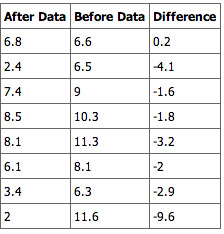Paired Samples Table 1: This table shows the before and after values of the data in our sample.

Are the sensory measurements, on average, lower after hypnotism? Test at a 5% significance level.

#### Solution

shows that the corresponding “before” and “after” values form matched pairs. (Calculate “after” minus “before”).Paired Samples Table 2: This table shows the before and after values and their calculated differences.

The data for the test are the differences:

{0.2, -4.1, -1.6, -1.8, -3.2, -2, -2.9, -9.6}

The sample mean and sample standard deviation of the differences are: \bar { { x }_{ d } } =-3.13 and

Verify these values. Let μd be the population mean for the differences. We use the subscript d to denote “differences”.

Random Variable: $\bar { { \text{x} }_{ \text{d} } }$ (the mean difference of the sensory measurements):

${ \text{H} }_{ 0 }:{ \mu }_{ \text{d} }\ge 0$

There is no improvement. ($\mu_\text{d}$ is the population mean of the differences.)

${ \text{H} }_{ \text{a} }:{ \mu }_{ \text{d} }<0$

There is improvement. The score should be lower after hypnotism, so the difference ought to be negative to indicate improvement.

Distribution for the test: The distribution is a student-$\text{t}$ with $\text{df} = \text{n}-1 = 8-1 = 7$. Use $\text{t}_7$. (Notice that the test is for a single population mean. )

Calculate the $\text{p}$-value using the Student-$\text{t}$ distribution: $\text{p}\text{-value} = 0.0095$

Graph: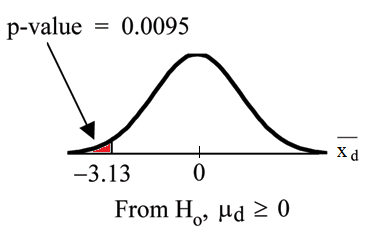$\text{p}$-Value Graph: This image shows the graph of the $\text{p}$-value obtained in our example.

$\bar { { \text{X} }_{ \text{d} } }$ is the random variable for the differences. The sample mean and sample standard deviation of the differences are:

$\bar { { \text{x} }_{ \text{d} } } =-3.13$

$\bar { { \text{s} }_{ \text{d} } } =2.91$

Compare $\alpha$ and the $\text{p}$-value: $\alpha = 0.05$ and $\text{p}\text{-value} = 0.0095$. $\alpha > \text{p}\text{-value}$.

Make a decision: Since $\alpha > \text{p}\text{-value}$, reject $\text{H}_0$. This means that $\mu_\text{d} < 0$, and there is improvement.

Conclusion: At a 5% level of significance, from the sample data, there is sufficient evidence to conclude that the sensory measurements, on average, are lower after hypnotism. Hypnotism appears to be effective in reducing pain.

## Comparing Two Population Variances

In order to compare two variances, we must use the $\text{F}$ distribution.

### Learning Objectives

Outline the $\text{F}$-test and how it is used to test two population variances.

### Key Takeaways

#### Key Points

• In order to perform a $\text{F}$ test of two variances, it is important that the following are true: (1) the populations from which the two samples are drawn are normally distributed, and (2) the two populations are independent of each other.
• When we are interested in comparing the two sample variances, we use the $\text{F}$ ratio: $\text{F}=\dfrac { \left[ \dfrac { { \text{s} }_{ 1 }^{ 2 } }{ { \sigma }_{ 1 }^{ 2 } } \right] }{ \left[ \dfrac { { \text{s} }_{ 2 }^{ 2 } }{ { \sigma }_{ 2 }^{ 2 } } \right] }$.
• If the null hypothesis is $\sigma_1^2 = \sigma_2^2$, then the $\text{F}$ ratio becomes: $\text{F}=\dfrac { \left[ \dfrac { { \text{s} }_{ 1 }^{ 2 } }{ { \sigma }_{ 1 }^{ 2 } } \right] }{ \left[ \dfrac { { \text{s} }_{ 2 }^{ 2 } }{ { \sigma }_{ 2 }^{ 2 } } \right] } =\dfrac { { \text{s} }_{ 1 }^{ 2 } }{ { \text{s} }_{ 2 }^{ 2 } }$.
• If the two populations have equal variances the $\text{F}$ ratio is close to 1.
• If the two population variances are far apart the $\text{F}$ ratio becomes a large number.
• Therefore, if $\text{F}$ is close to 1, the evidence favors the null hypothesis (the two population variances are equal); but if $\text{F}$ is much larger than 1, then the evidence is against the null hypothesis.

#### Key Terms

• null hypothesis: A hypothesis set up to be refuted in order to support an alternative hypothesis; presumed true until statistical evidence in the form of a hypothesis test indicates otherwise.
• F distribution: A probability distribution of the ratio of two variables, each with a chi-square distribution; used in analysis of variance, especially in the significance testing of a correlation coefficient ($\text{R}$ squared).

It is often desirable to compare two variances, rather than two means or two proportions. For instance, college administrators would like two college professors grading exams to have the same variation in their grading. In order for a lid to fit a container, the variation in the lid and the container should be the same. A supermarket might be interested in the variability of check-out times for two checkers. In order to compare two variances, we must use the $\text{F}$ distribution.

In order to perform a $\text{F}$ test of two variances, it is important that the following are true:

1. The populations from which the two samples are drawn are normally distributed.
2. The two populations are independent of each other.

Suppose we sample randomly from two independent normal populations. Let $\sigma_1^2$ and $\sigma_2^2$ be the population variances and $\text{s}_1^2$ and $\text{s}_2^2$ be the sample variances. Let the sample sizes be $\text{n}_1$ and $\text{n}_2$. Since we are interested in comparing the two sample variances, we use the $\text{F}$ ratio:

$\text{F}=\dfrac { \left[ \dfrac { { \text{s} }_{ 1 }^{ 2 } }{ { \sigma }_{ 1 }^{ 2 } } \right] }{ \left[ \dfrac { { \text{s} }_{ 2 }^{ 2 } }{ { \sigma }_{ 2 }^{ 2 } } \right] }$

$\text{F}$ has the distribution $\text{F} \sim \text{F}(\text{n}_1 - 1, \text{n}_2 - 1)$ where $\text{n}_1 - 1$ are the degrees of freedom for the numerator and $\text{n}_2 - 1$ are the degrees of freedom for the denominator.

If the null hypothesis is $\sigma_1^2 = \sigma_2^2$, then the $\text{F}$ ratio becomes:

$\text{F}=\dfrac { \left[ \dfrac { { \text{s} }_{ 1 }^{ 2 } }{ { \sigma }_{ 1 }^{ 2 } } \right] }{ \left[ \dfrac { { \text{s} }_{ 2 }^{ 2 } }{ { \sigma }_{ 2 }^{ 2 } } \right] } =\dfrac { { \text{s} }_{ 1 }^{ 2 } }{ { \text{s} }_{ 2 }^{ 2 } }$

Note that the $\text{F}$ ratio could also be $\frac { { \text{s} }_{ 2 }^{ 2 } }{ { \text{s} }_{ 1 }^{ 2 } }$. It depends on $\text{H}_\text{a}$ and on which sample variance is larger.

If the two populations have equal variances, then $\text{s}_1^2$ and $\text{s}_2^2$ are close in value and $\text{F}=\frac { { \text{s} }_{ 1 }^{ 2 } }{ { \text{s} }_{ 2 }^{ 2 } }$ is close to 1. But if the two population variances are very different, $\text{s}_1^2$ and $\text{s}_2^2$ tend to be very different, too. Choosing $\text{s}_1^2$ as the larger sample variance causes the ratio $\frac { { \text{s} }_{ 1 }^{ 2 } }{ { \text{s} }_{ 2 }^{ 2 } }$ to be greater than 1. If $\text{s}_1^2$ and $\text{s}_2^2$ are far apart, then $\text{F}=\frac { { \text{s} }_{ 1 }^{ 2 } }{ { \text{s} }_{ 2 }^{ 2 } }$ is a large number.

Therefore, if $\text{F}$ is close to 1, the evidence favors the null hypothesis (the two population variances are equal). But if $\text{F}$ is much larger than 1, then the evidence is against the null hypothesis.

A test of two variances may be left, right, or two-tailed.

### Example

Two college instructors are interested in whether or not there is any variation in the way they grade math exams. They each grade the same set of 30 exams. The first instructor’s grades have a variance of 52.3. The second instructor’s grades have a variance of 89.9.

Test the claim that the first instructor’s variance is smaller. (In most colleges, it is desirable for the variances of exam grades to be nearly the same among instructors.) The level of significance is 10%.

#### Solution

Let 1 and 2 be the subscripts that indicate the first and second instructor, respectively: $\text{n}_1 = \text{n}_2 = 30$.

$\text{H}_0: \sigma_1^2 = \sigma_2^2$ and $\text{H}_\text{a}: \sigma_1^2 < \sigma_2^2$

Calculate the test statistic: By the null hypothesis ($\sigma_1^2 = \sigma_2^2$), the F statistic is:

$\displaystyle \text{F}=\frac { \left[ \frac { { \text{s} }_{ 1 }^{ 2 } }{ { \sigma }_{ 1 }^{ 2 } } \right] }{ \left[ \frac { { \text{s} }_{ 2 }^{ 2 } }{ { \sigma }_{ 2 }^{ 2 } } \right] } =\frac { { \text{s} }_{ 1 }^{ 2 } }{ { \text{s} }_{ 2 }^{ 2 } } =\frac { 52.3 }{ 89.9 } =0.6$

Distribution for the test: $\text{F}_{29, 29}$ where $\text{n}_1-1 = 29$ and $\text{n}_2 -1 = 29$.

Graph: This test is left-tailed: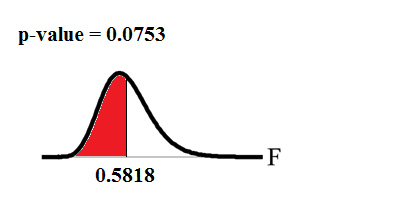$\text{p}$-Value Graph: This image shows the graph of the $\text{p}$-value we calculate in our example.

Probability statement: $\text{p}\text{-value} = \text{P}(\text{F}<0.5818) = 0.0753$.

Compare $\alpha$ and the $\text{p}$-value: $\alpha = 0.10 > \text{p}\text{-value}$.

Make a decision: Since $\alpha > \text{p}\text{-value}$, reject $\text{H}_0$.

Conclusion: With a 10% level of significance, from the data, there is sufficient evidence to conclude that the variance in grades for the first instructor is smaller.

## Determining Sample Size

A common problem is calculating the sample size required to yield a certain power for a test, given a predetermined type I error rate $\alpha$.

### Learning Objectives

Calculate the appropriate sample size required to yield a certain power for a hypothesis test by using predetermined tables, Mead’s resource equation or the cumulative distribution function.

### Key Takeaways

#### Key Points

• In a hypothesis test, sample size can be estimated by pre-determined tables for certain values, by Mead’s resource equation, or, more generally, by the cumulative distribution function.
• Using desired statistical power and Cohen’s $\text{D}$ in a table can yield an appropriate sample size for a hypothesis test.
• Mead’s equation may not be as accurate as using other methods in estimating sample size, but gives a hint of what is the appropriate sample size where parameters such as expected standard deviations or expected differences in values between groups are unknown or very hard to estimate.
• In a hypothesis test, sample size can be estimated by pre-determined tables for certain values, by Mead’s resource equation, or, more generally, by the cumulative distribution function.

#### Key Terms

• Mead’s resource equation: $\text{E}=\text{N}-\text{B}-\text{T}$: an equation that gives a hint of what the appropriate sample size is, where parameters such as expected standard deviations or expected differences in values between groups are unknown or very hard to estimate.
• Cohen’s D: A measure of effect size indicating the amount of different between two groups on a construct of interest in standard deviation units.

### Required Sample Sizes for Hypothesis Tests

A common problem faced by statisticians is calculating the sample size required to yield a certain power for a test, given a predetermined Type I error rate $\alpha$. As follows, this can be estimated by pre-determined tables for certain values, by Mead’s resource equation, or, more generally, by the cumulative distribution function.

### By Tables

The table shown in can be used in a two-sample $\text{t}$-test to estimate the sample sizes of an experimental group and a control group that are of equal size—that is, the total number of individuals in the trial is twice that of the number given, and the desired significance level is 0.05.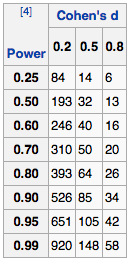Sample Size Determination: This table can be used in a two-sample $\text{t}$-test to estimate the sample sizes of an experimental group and a control group that are of equal size.

The parameters used are:

• The desired statistical power of the trial, shown in column to the left.
• Cohen’s $\text{D}$ (effect size), which is the expected difference between the means of the target values between the experimental group and the control group divided by the expected standard deviation.

Mead’s resource equation is often used for estimating sample sizes of laboratory animals, as well as in many other laboratory experiments. It may not be as accurate as using other methods in estimating sample size, but gives a hint of what is the appropriate sample size where parameters such as expected standard deviations or expected differences in values between groups are unknown or very hard to estimate.

All the parameters in the equation are in fact the degrees of freedom of the number of their concepts, and hence, their numbers are subtracted by 1 before insertion into the equation. The equation is:

$\text{E}=\text{N}-\text{B}-\text{T}$

where:

• $\text{N}$ is the total number of individuals or units in the study (minus 1)
• $\text{B}$ is the blocking component, representing environmental effects allowed for in the design (minus 1)
• $\text{T}$ is the treatment component, corresponding to the number of treatment groups (including control group) being used, or the number of questions being asked (minus 1)
• $\text{E}$ is the degrees of freedom of the error component, and should be somewhere between 10 and 20.

### By Cumulative Distribution Function

Let $\text{X}_\text{i}, \text{i} = 1, 2, \dots, \text{n}$, be independent observations taken from a normal distribution with unknown mean $\mu$ and known variance $\sigma^2$. Let us consider two hypotheses, a null hypothesis:

$\text{H}_0: \mu = 0$

and an alternative hypothesis:

$\text{H}_\text{a}: \mu = \mu^*$

for some “smallest significant difference” $\mu^* > 0$. This is the smallest value for which we care about observing a difference. Now, if we wish to:

1. reject $\text{H}_0$ with a probability of at least $1-\beta$ when $\text{H}_\text{a}$ is true (i.e., a power of $1-\beta$), and
2. reject $\text{H}_0$ with probability $\alpha$ when $\text{H}_0$ is true,

then we need the following:

If $\text{z}_{\alpha}$ is the upper $\alpha$ percentage point of the standard normal distribution, then:

$\displaystyle \text{Pr}\left( \frac { \bar { \text{x} } >{ \text{z} }_{ \text{a} }\sigma }{ \sqrt { \text{n} } } |{ \text{H} }_{ 0 } \ \text{ is true} \right) =\alpha$,

and so “reject $\text{H}_0$ if our sample average is more than $\frac { { \text{z} }_{ \text{a} }\sigma }{ \sqrt { \text{n} } }$” is a decision rule that satisfies number 2 above. Note that this is a one-tailed test.